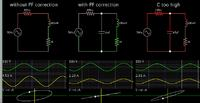problem in inductive current measrement with acs712 hall effect sensor

1. problem in inductive current measrement with acs712 hall effect sensor

I am having problem in measuring inductive load current with acs712(20amps) hall effect sensor, for resistive load it works 99% accurately. I am using pic micr 18f4620 this is my sample code

 Code C - [expand] 1 2 3 4 5 6 7 8 9 10 11 12 13 14 15 16 17 18 19 20 21 22 23 24 25 26 27 28 29 30 31 32 33 34 35 36 37 38 39 40 41 42 43 While(1) { n1 = 0;        j = 0; for(int16 i = 0;i<20;i++) { n1++;   Table_sinusoide[j] =get_adc_value(3); delay_us(997); j++; } mini = 1023; maxi =1; for(j=0;jM) { mini=M; } if(maxi

my idea is simple for 20 ms time(50hz line freq) I am taking 20 samples the finding min and max values
p2p = maxi-mini.
I am getting exact result for resistive loads, my hall effect sensor produces 100mv / amp
For inductive loads such as motors the value shown is In accurate, I also increased the sampling rate for 20ms I took 600 samples but the result is same. for 4 amps motor load my hall effect sensor outputs 400mv measured in multimeter but in pic I am getting in accurate results why so kindly help I want to measure accurate ac current for both inductive and resistive loads.
Regards
Kalyan.•

2. Re: problem in inductive current measrement with acs712 hall effect sensorOriginally Posted by raman00084I am having problem in measuring inductive load current
Could this be a power factor issue? An inductive load tries to draw excessively high Amperes during low times in the voltage waveform.

When you apply power factor correction, you reduce Ampere draw.

Simulation demo:1 members found this post helpful.--[[ ]]--